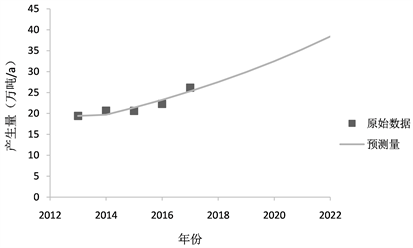﻿ 工业危险废物预测及管控对策研究

# 工业危险废物预测及管控对策研究Research on Industrial Hazardous Waste Prediction and Control Countermeasures

Abstract: With the rapid development of the social economy and industry, the amount of industrial hazardous waste has increased dramatically. Through the establishment of the GM(1, 1) model, the amount of industrial hazardous waste generated in Chengdu in the next few years is predicted. The results show that the amount of industrial hazardous waste generated in Chengdu is expected to reach 383,000 tons by 2022, and the countermeasures for the control of hazardous wastes are proposed.

1. 引言

2. 文献综述

3. 模型方法

3.1. 模型介绍

GM(1, 1)模型是一种灰色模型，GM(1, 1)模型其实是GM(1, N)模型中N = 1的特例，即建立的模型中只包含一个变量，并且微分方程是一阶的，它是对离散序列建立的微分方程  。

${X}^{\left(1\right)}=\left({x}^{\left(1\right)}\left(1\right),{x}^{\left(1\right)}\left(2\right),\cdots ,{x}^{\left(1\right)}\left(n\right)\right)$

${X}^{\left(1\right)}$ 的紧邻均值生成序列为：

${Z}^{\left(1\right)}=\left({z}^{\left(1\right)}\left(1\right),{z}^{\left(1\right)}\left(2\right),\cdots ,{z}^{\left(1\right)}\left(n\right)\right)$

${z}^{\left(1\right)}\left(k\right)=0.5\ast \left({x}^{\left(1\right)}\left(k\right)+{x}^{\left(1\right)}\left(k-1\right)\right),k=2,3,\cdots ,n.$

$\frac{d{X}^{\left(1\right)}}{dt}+a{X}^{\left(1\right)}=b$

$\stackrel{^}{a}={\left(a,b\right)}^{T}={\left({B}^{T}B\right)}^{-1}{B}^{T}{y}_{n}$

$B=\left[\begin{array}{cc}\begin{array}{c}-{z}^{\left(1\right)}\left(2\right)\\ -{z}^{\left(1\right)}\left(3\right)\end{array}& \begin{array}{c}1\\ 1\end{array}\\ \begin{array}{c}⋮\\ -{z}^{\left(1\right)}\left(n\right)\end{array}& \begin{array}{c}⋮\\ 1\end{array}\end{array}\right],{y}_{n}=\left[\begin{array}{c}\begin{array}{c}{x}^{\left(0\right)}\left(2\right)\\ {x}^{\left(0\right)}\left(3\right)\end{array}\\ \begin{array}{c}⋮\\ {x}^{\left(0\right)}\left(n\right)\end{array}\end{array}\right]$

${\stackrel{^}{x}}^{\left(1\right)}\left(n+1\right)=\left({X}^{\left(0\right)}\left(1\right)-\frac{b}{a}\right){e}^{-an}+\frac{b}{a}$

${\stackrel{^}{x}}^{\left(1\right)}$ 实行累减生成预测值：

${\stackrel{^}{x}}^{\left(0\right)}\left(n+1\right)={\stackrel{^}{x}}^{\left(1\right)}\left(n+1\right)-{\stackrel{^}{x}}^{\left(1\right)}\left(n\right)$

3.2. 精度检验

${S}_{1}=\sqrt{\frac{{\sum }^{\text{​}}{\left[{X}^{\left(0\right)\left(n\right)}-{\stackrel{¯}{x}}^{\left(0\right)}\right]}^{2}}{n-1}}$

${S}_{2}=\sqrt{\frac{{\sum }^{\text{​}}{\left[{\epsilon }^{\left(1\right)}\left(n\right)-{\stackrel{¯}{\epsilon }}^{\left(1\right)}\right]}^{2}}{n-1}}$Table 1. Post-test difference accuracy standard table

4. 数据来源Table 2. Industrial hazardous waste production in Chengdu, 2013-2017

*数据来源：《成都市固体废物污染环境防治信息公告》

5. 预测结果及分析

2018~2022年成都市工业危险废物产生量预测结果见表3Table 3. Forecast Results of Industrial Hazardous Waste Generation in Chengdu from 2018 to 2022Figure 1. Curve of industrial hazardous waste production in Chengdu

6. 危险废物管理对策研究

1) 提升危险废物综合利用能力，对产生的工业危险废物应优先鼓励“资源化”，以市场需求为导向，推进危险废物综合利用设施建设。对已建危险废物综合利用经营单位鼓励企业逐步进行改扩建工程增加综合利用规模，对我市危险废物综合利用缺口较大且暂无综合利用设施的，鼓励社会资本参与建设。

2) 加大企业对危险废物减量化力度，目前我国工业企业内部危险废物减量化设施较少，产废企业对外依赖性较强，企业源头减量的主体责任落实不到位。应鼓励产废单位自行或寻找第三方机构制定企业危险废物减量化方案，危险废物产废单位应据此方案开展源头削减工作。

3) 加快危废处置经济政策研究制定，研究制定有利于促进危险废物减量化、资源化的经济政策。积极探索危险废物利用及处理“以奖促治”制度，在财税、价格等政策方面扶持危险废物利用处置行业发展。进一步落实和完善工业危险废物处置收费制度，督促产生单位将危险废物处置费用纳入企业生产运营支出预算中。

4) 推广危险废物处置利用新技术，针对危险废物经营单位，应大力借鉴国内先进地区危险废物处置利用新技术新工艺，提升企业运营管理水平，提高处置处理水平。

7. 结论

 王琪, 黄启飞, 闫大海, 等. 我国危险废物管理的现状与建议[J]. 环境工程技术学报, 2013, 3(1): 1-5.

 李金惠, 聂永丰, 白庆中. 中国工业固体废物产生量预测研究[J]. 环境科学学报, 1999, 19(6): 626-630.

 林艺芸, 张江山, 刘常青, 等. 我国工业危险废物产生现状及产量预测[J]. 有色制金与设计, 2007(Z1): 18-21.

 郭平, 王京刚, 周炳炎. 我国工业危险废物产生量的预测研究[J]. 环境科学与技术, 2006, 29(2): 56-57.

 周炳炎, 郭平, 王琪, 等. 北京市工业危险废物产生特性研究[J]. 环境科学与管理, 2006, 31(1): 40-43.

 郑炜. 福建省工业危险废物产生趋势预测与对策[J]. 化学工程与装备, 2018, 260(9): 323-324.

 刘思峰. 灰色系统理论的产生与发展[J]. 南京航空航天大学学报, 2004, 36(4): 267-271.

Top